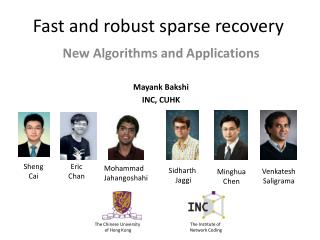Download PresentationFast and robust sparse recoveryFast and robust sparse recovery - PowerPoint PPT Presentation

Download PresentationFast and robust sparse recovery
An Image/Link below is provided (as is) to download presentation

Download Policy: Content on the Website is provided to you AS IS for your information and personal use and may not be sold / licensed / shared on other websites without getting consent from its author. While downloading, if for some reason you are not able to download a presentation, the publisher may have deleted the file from their server.

- - - - - - - - - - - - - - - - - - - - - - - - - - - E N D - - - - - - - - - - - - - - - - - - - - - - - - - - -
Presentation Transcript

1. Fast and robust sparse recovery Mayank Bakshi INC, CUHK New Algorithms and Applications Sheng Cai Eric Chan Mohammad Jahangoshahi Sidharth Jaggi Venkatesh Saligrama Minghua Chen The Chinese University of Hong Kong The Institute of Network Coding

2. Fast and robust sparse recovery m ? n Measurement Measurement output k m<n Reconstruct x Unknown x

3. A. Compressive sensing ? m ? n k k ≤ m<n

4. A. Robust compressive sensing ? e z y=A(x+z)+e Approximate sparsity Measurement noise

5. Computerized Axial Tomography (CAT scan)

6. B. Tomography y = Tx Estimate x given y and T

7. B. Network Tomography • Transform T: • Network connectivity matrix (known a priori) • Measurements y: • End-to-end packet delays • Infer x: • Link/node congestion Hopefully “k-sparse” Compressive sensing? • Challenge: • Matrix T “fixed” • Can only take “some” • types of measurements

8. n-d C. Robust group testing d q 0 1 q 0 1 For Pr(error)< ε , Lower bound: What’s known …[CCJS11] Noisy Combinatorial OMP:

9. A. Robust compressive sensing ? e z y=A(x+z)+e Approximate sparsity Measurement noise

10. Apps: 1. Compression W(x+z) x+z BW(x+z) = A(x+z) M.A. Davenport, M.F. Duarte, Y.C. Eldar, and G. Kutyniok, "Introduction to Compressed Sensing,"inCompressed Sensing: Theory and Applications, 2012

11. Apps: 2. Fast(er) Fourier Transform H. Hassanieh, P. Indyk, D. Katabi, and E. Price. Nearly optimal sparse fourier transform. InProceedings of the 44th symposium on Theory of Computing (STOC '12).

12. Apps: 3. One-pixel camera http://dsp.rice.edu/sites/dsp.rice.edu/files/cs/cscam.gif

13. y=A(x+z)+e

14. y=A(x+z)+e

15. y=A(x+z)+e

16. y=A(x+z)+e

17. y=A(x+z)+e (Information-theoretically) order-optimal

18. (Information-theoretically) order-optimal • Support Recovery

19. SHO-FA:SHO(rt)-FA(st)

20. O(k) measurements, O(k) time

21. 1. Graph-Matrix A d=3 ck n

22. 1. Graph-Matrix A d=3 ck n

23. 1. Graph-Matrix

24. 2. (Most) x-expansion ≥2|S| |S|

25. 3. “Many” leafs L+L’≥2|S| ≥2|S| |S| 3|S|≥L+2L’ L≥|S| L+L’≤3|S| L/(L+L’) ≥1/2 L/(L+L’) ≥1/3

26. 4. Matrix

27. Encoding – Recap. 0 1 0 1 0

28. Decoding – Initialization

29. Decoding – Leaf Check(2-Failed-ID)

30. Decoding – Leaf Check (4-Failed-VER)

31. Decoding – Leaf Check(1-Passed)

32. Decoding – Step 4 (4-Passed/STOP)

33. Decoding – Recap. 0 0 0 0 0 0 0 0 1 0 ? ? ?

34. Decoding – Recap. 0 1 0 1 0

35. Noise/approx. sparsity

36. Meas/phase error

37. Correlated phase meas.

38. Correlated phase meas.

39. Correlated phase meas.

40. Network Tomography • Goal: Infer network characteristics (edge or node delay) • Difficulties: • Edge-by-edge (or node-by node) monitoring too slow • Inaccessible nodes

41. Network Tomography • Goal: Infer network characteristics (edge or node delay) • Difficulties: • Edge-by-edge (or node-by node) monitoring too slow • Inaccessible nodes • Network Tomography: • with very fewend-to-end measurements • quickly • for arbitrary network topology

42. B. Network Tomography • Transform T: • Network connectivity matrix • (known a priori) • Measurements y: • End-to-end packet delays • Infer x: • Link/node congestion Hopefully “k-sparse” Our algorithm: FRANTIC Compressive sensing? • Challenge: • Matrix T “fixed” • Can only take “some” • types of measurements • Fast Reference-based Algorithm for Network Tomography vIa Compressive sensing • Idea: • “Mimic” random matrix

43. SHO-FA A d=3 ck n

44. 2. Better mimicking of desired T

45. Node delay estimation

46. Node delay estimation

47. Node delay estimation

48. Edge delay estimation